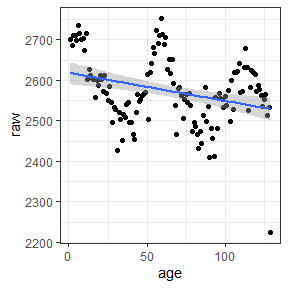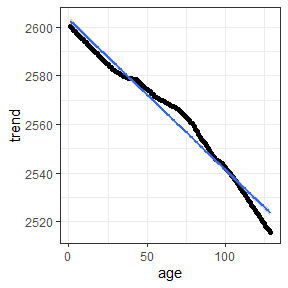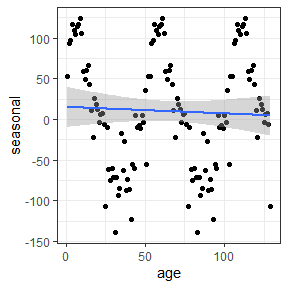# Feature Correction

Occasionally, PV data can include features such as saturation, failure, or strong seasonality that should be removed prior or during examination. This package includes feature correction methods addressing each of these.

## Saturation Removal

Inverter saturation can throw off trends in the data as well. If you know the saturation limit of the inverter for your data, you can run this function to remove saturated data points.

test_dfc_removed_saturation <- plr_saturation_removal(test_dfc, var_list, sat_limit = 3000, power_thresh = 0.99)

# fraction of data kept
nrow(test_dfc_removed_saturation)/nrow(test_dfc)
#>  0.994224

## System Failures and Soiling

If a user knows or suspects that a period of system failure is present in the data, this package offers solutions for identifying and removing these data points. The method plr_failure_test filters data for particularly low correlation between power and irradiance, and then executes k-means clustering (with k=2) to identify a cluster of points which may indicate soiling. It has an option to group data by months or look over all data. The function removes data which is in the smaller cluster if the cluster indicates much lower power production per irradiance and accounts for a small (<.25) portion of the data.

# default values inserted for reference
#df_failure <- plr_failure_test(test_dfc, var_list, corr_thresh = 0.95, plot = FALSE, by_month = FALSE)

# fraction of data kept
#nrow(df_failure)/nrow(test_dfc)

The method includes an option to plot slopes of day-by-day linear models of irradiance and power production against days. In order to plot, one must specify a file_path and file_name to save the plot under; it is not returned within the R environment. The generated boxplot is of those same slopes, visually identifying outliers which may represent failures or soiling.

## Seasonality Decomposition

Following power prediction, seasonality may still be apparent in the data. This is often the case in the XbX model, the data-driven nature of which is prone to leaving in seasonality. Decomposition, the statistical method of removing seasonality from data, can be performed on such power predicted data.

test_xbx_wbw_decomp <- plr_decomposition(test_xbx_wbw_res, freq = 52, power_var = 'power_var', time_var = 'time_var', plot = FALSE, plot_file = NULL, title = NULL, data_file = NULL)

# generate a pretty table
knitr::kable(test_xbx_wbw_decomp[1:5, ], caption = "XbX Week-by-Week Decomposition: Resulting Data")
XbX Week-by-Week Decomposition: Resulting Data
raw seasonal trend remainder weights sub.labels interpolated age sigma operating power
2699.661 53.13425 2600.062 46.465313 1 subseries 1 FALSE 1 64.56543 1 2600.062
2686.040 93.35386 2599.413 -6.727034 1 subseries 2 FALSE 2 33.34219 2 2599.413
2711.840 96.67574 2598.748 16.415820 1 subseries 3 FALSE 3 33.58805 3 2598.748
2709.884 117.10601 2598.075 -5.296855 1 subseries 4 FALSE 4 34.55537 4 2598.075
2697.696 109.10639 2597.400 -8.809981 1 subseries 5 FALSE 5 49.33841 5 2597.400
# make plots of the decomposed data
raw_plot <- ggplot2::ggplot(test_xbx_wbw_decomp, aes(age, raw)) +
geom_point() +
geom_smooth( method = "lm") +
theme_bw()

trend_plot <- ggplot2::ggplot(test_xbx_wbw_decomp, aes(age, trend)) +
geom_point() +
geom_smooth( method = "lm") +
theme_bw()

seasonal_plot <- ggplot2::ggplot(test_xbx_wbw_decomp, aes(age, seasonal)) +
geom_point() +
geom_smooth( method = "lm") +
theme_bw()

raw_plot
#> geom_smooth() using formula 'y ~ x'trend_plot
#> geom_smooth() using formula 'y ~ x'seasonal_plot
#> geom_smooth() using formula 'y ~ x'